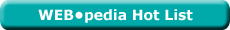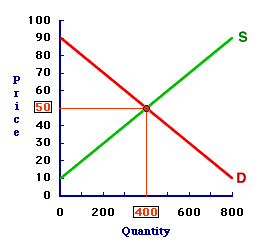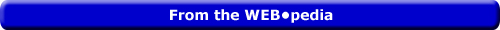Friday  March 22, 2019
 AmosWEB means Economics with a Touch of Whimsy!YTM: The common abbreviation for yield to maturity, which is the annual rate of return on a financial asset that is held until maturity. Yield to maturity depends on both the coupon rate and the face or par value paid at maturity. If the selling price of a financial asset is equal to its par value, then the yield to maturity is equal to the current yield and the coupon rate. However, if the asset is selling at a discount, then the yield to maturity exceeds the current yield, which is greater than the coupon rate. And if the asset is selling at a premium, then the yield to maturity is less than the current yield, which is below than the coupon rate.Most Viewed (Number) Visit the WEB*pediaLesson 7: Market | Unit 3: A Graph Page: 11 of 22

 Topic: The Equilibrium <=PAGE BACK | PAGE NEXT=>This market graph combines the forces of demand and supply.
• Equilibrium is achieved as each side of the market seeks a mutually agreeable exchange.
• The equilibrium price is 50 cents and the equilibrium quantity is 400 tapes. At this 50-price, the quantity demanded is equal to the quantity supplied.
• At no other price is the quantity demanded and supplied equal.
• That's equilibrium.

| | |ELASTICITY AND SUPPLY INTERCEPT

The intersection of a straight-line supply curve with vertical price axis and/or horizontal quantity axis reveals the relative price elasticity of supply. Intersection with the horizontal quantity axis means inelastic and intersection with the vertical price axis means elastic. Intersection with the origin means unit elastic supply.BLUE PLACIDOLA[What's This?] Today, you are likely to spend a great deal of time at a dollar discount store looking to buy either several magazines on fashion design or a package of 3 by 5 index cards, the ones without lines. Be on the lookout for jovial bank tellers.Your Complete ScopeMore money is spent on gardening than on any other hobby."Most of the things worth doing in the world had been declared impossible before they were done."-- Louis D. Brandeis, Supreme Court JusticeFVFace ValueA PEDestrian's Guide Xtra CreditTell us what you think about AmosWEB. Like what you see? Have suggestions for improvements? Let us know. Click the User Feedback link.| | | | | | | | | | |
| | | |

Thanks for visiting AmosWEB